## Kaggle’s “Human or Robot?” competition feedbackA month ago, I started participating in my first Kaggle competition. I wanted to start participating in Kaggle competitions for a while and Facebook launched a recruiting competition: Human or Robot?, and I decided to join the party.

I was finally ranked 32nd on the final ranking (private learderboard), and I could actually have been ranked 17th if I had chosen another of my predictions as final submission… (That additionnal URL feature wasn’t that useless afterall…)

This blog post is a (slightly modified) export of my IPython notebooks written for this competition. Well… Let’s get started!

TL;DR: I used a bunch of basic statistical features extracted from aggregation of bids of each bidder and trained a Random Forest tuned by 10-fold-CV. Plain and simple.

# Import needed libraries for dataset creation

Nothing fancy here, we just use some classical Python Data Science libraries: numpy, scikit-learn and pandas, plus pickle to save the result.

import pickle
import numpy as np
import sklearn.preprocessing
from sklearn_pandas import DataFrameMapper
import pandas as pd


# Read the data

First step is to read the CSV files and load them as Pandas frames. I used Pandas here because of the “heavy” work needed to create the features, being very easy to do with Pandas and that would have been much more painful to do with numpy only for example.

# Read the bids, replace NaN values with a dash because NaN are string categories we don't know
bids = pd.read_csv('bids.csv', header=0)
bids.fillna('-', inplace=True)

# Load train and test bidders lists (nothing really interesting in that list)
train = pd.read_csv('train.csv', header=0, index_col=0)
test = pd.read_csv('test.csv', header=0, index_col=0)

# Join those 2 datasets together (-1 outcome meaning unknown i.e. test)
test['outcome'] = -1.0
bidders = pd.concat((train, test))


# Dataset investigation

Prior to the feature creation, some inspection on the data has been done, of course, but I didn’t kept any trace of this quick’n’dirty work anywhere and won’t be able to show it to you.

The idea is to get to know what’s in the dataset. The first step is just to show look at the raw data, and to then look at some stats about the data. For example, the first thing you might wonder is what the heck can I do with those payment_account and address hashes that Kaggle gave me for each bidder. Is there any of these things that appear more than once? Nope, they are as unique as the bidder_id so you can throw this away. At this moment you know that all your features must come from the bids themselves.

Then you do some stats and plots of what’s in the bids, you look what could be interesting to compute features…

# Features creation

… and then there is a moment when you decide that it’s time to get this party started and start to do something with these bids.

The things I hesitated about was to decide if I should predict if a bidder is a bot based on aggregates of info about his bids, or if I should predict if a bid has been made by a bot for each bid and then aggregate the predictions at bid level to create a prediction at bidder level.

I preferred to work at the bidder level because I had the feeling that each bid don’t have enough info by itself to allow proper prediction, and that the aggregation of a set of bids would allow me to create more high level features about the general behavior of a bidder and therefore get more useful info.

I didn’t have the time to actually try the bid-level prediction approach. I don’t know how it would have turned out.

So the first thing you do is to look at the info you have on each bid and look at what feature you can compute with this. We have this:

• auction (category) – Unique identifier of an auction
• merchandise (category) – The category of the auction site campaign, which means the bidder might come to this site by way of searching for “home goods” but ended up bidding for “sporting goods” – and that leads to this field being “home goods”. This categorical field could be a search term, or online advertisement.
• device (category) – Phone model of a visitor
• time (real) – Time that the bid is made (transformed to protect privacy).
• country (category) – The country that the IP belongs to
• ip (category) – IP address of a bidder (obfuscated to protect privacy).
• url (category) – url where the bidder was referred from (obfuscated to protect privacy).

We only have one real feature and lots of categories.

My first approach was what I’ve seen called “kitchen sink approach”, I basically decided to compute whatever statistical computation crossed my mind (as long as I wasn’t too lazy to implement it so it had better be simple or genius). I decided to apply the same analysis on all categories. And the same idea goes for the real variable, with different stats of course.

## Category variable feature extraction

The idea is to group the bids of a bidder and compute stats about the group (which is therefore a series of value for each variable). Then for a list of string, you get stats about these strings and their frequencies:

• number of unique categories that appear
• highest frequency that appearance
• lowest frequency of appearance
• category that appear the most
• standard deviation of the frequencies

and… that’s it!

def computeStatsCat(series, normalizeCount = 1.0):

n = float(series.shape)
counts = series.value_counts()

nbUnique = counts.count() / normalizeCount
hiFreq = counts / n
loFreq = counts[-1] / n
argmax = counts.index
stdFreq = np.std(counts / n)

return (nbUnique, loFreq, hiFreq, stdFreq, argmax)


## Real variable feature extraction

For time, I decided to go a little bit deeper and group the series of timestamp of bids by auction and have two stages of stats, stats at auction level that are then aggregated and global stats for the whole set of timestamps of the bidder.

You see below a few functions that allowed me to compute those features. Basically, for each auction I compute stats that are: min, max, range of timestamps, and then I compute a bunch of things about interval between two bids: mean interval, standard deviation, percentiles. I then aggregate those results for all the auction a bidder had always in the same ideas of simple stats.

# Compute stats of numerical series without caring about interval between values
def computeStatsNumNoIntervals(series):

min = series.min()
max = series.max()
mean = np.mean(series)
std = np.std(series)
perc20 = np.percentile(series, 20)
perc50 = np.percentile(series, 50)
perc80 = np.percentile(series, 80)

return (min, max, mean, std, perc20, perc50, perc80)

# Compute stats of a numerical series, taking intervals between values into account
def computeStatsNum(series, copy = True):

if copy:
series = series.copy()

series.sort()
intervals = series[1:].as_matrix() - series[:-1].as_matrix()
if len(intervals) < 1:
intervals = np.array()

nb = series.shape
min = series.min()
max = series.max()
range = max - min
intervalsMin = np.min(intervals)
intervalsMax = np.max(intervals)
intervalsMean = np.mean(intervals)
intervalsStd = np.std(intervals)
intervals25 = np.percentile(intervals, 25)
intervals50 = np.percentile(intervals, 50)
intervals75 = np.percentile(intervals, 75)

return (nb, min, max, range,
intervalsMin, intervalsMax, intervalsMean, intervalsStd,
intervals25, intervals50, intervals75)

# Compute stats about a numerical column of table, with stats on sub-groups of this column (auctions in our case).
def computeStatsNumWithGroupBy(table, column, groupby):

# get series and groups
series = table[column]
groups = table.groupby(groupby)

# global stats
(nb, min, max, range,
intervalsMin, intervalsMax, intervalsMean, intervalsStd,
intervals25, intervals50, intervals75) = computeStatsNum(series)

# stats by group
X = []
for _, group in groups:
(grpNb, _, _, grpRange, grpIntervalsMin, grpIntervalsMax, grpIntervalsMean, grpIntervalsStd, _, _, _) = computeStatsNum(group[column])
X.append([grpNb, grpRange, grpIntervalsMin, grpIntervalsMax, grpIntervalsMean, grpIntervalsStd])
X = np.array(X)

grpNbMean = np.mean(X[:,0])
grpNbStd = np.std(X[:,0])
grpRangeMean = np.mean(X[:,1])
grpRangeStd = np.std(X[:,1])
grpIntervalsMinMin = np.min(X[:,2])
grpIntervalsMinMean = np.mean(X[:,2])
grpIntervalsMaxMax = np.max(X[:,3])
grpIntervalsMaxMean = np.mean(X[:,3])
grpIntervalsMean = np.mean(X[:,4])
grpIntervalsMeanStd = np.std(X[:,4])
grpIntervalsStd = np.mean(X[:,5])

return (nb, min, max, range,
intervalsMin, intervalsMax, intervalsMean, intervalsStd,
intervals25, intervals50, intervals75,
grpNbMean, grpNbStd, grpRangeMean, grpRangeStd,
grpIntervalsMinMin, grpIntervalsMinMean, grpIntervalsMaxMax, grpIntervalsMaxMean,
grpIntervalsMean, grpIntervalsMeanStd, grpIntervalsStd)


## Feature tried that did not really worked

### From categories to real values

In a desperate attempt to increase my score, I though about replacing categories in the bids dataset by real values by computing general stats about each category of each variable, and replacing this category by stats about this category, in my case the probability of this category to belong to appear in a bot’s bid.

I am aware that this is getting close to the danger of Data Leakage because you are explicitly introducing information about the target in the features. However, I feel like because the real value you use to represent the category is computed on the whole dataset, it might in some cases be ok because it is a very aggregated info, provided you have a lot of data in each category.

In this case, I think that if was definitely a data leakage because I got a 0.97 AUC on my CV but a 0.86 score on public leaderboard (a big drop from my results without those features). But it was worth trying!

def computeOutcomeProbaByCat(data, cats):
stats = {}
for cat in cats:
stats[cat] = pd.DataFrame(data.groupby(cat).aggregate(np.mean).outcome)
stats[cat].rename(columns={'outcome': cat+'Num'}, inplace=True)
return stats

#bidsWithOutcome = pd.merge(bids, bidders[['outcome']], how='left', left_on='bidder_id', right_index=True)
#stats = computeOutcomeProbaByCat(bidsWithOutcome[bidsWithOutcome.outcome >= 0], [u'auction', u'merchandise', u'device', u'country', u'url'])

# Add real columns to bids dataframe
#for cat in stats:
#    bids = pd.merge(bids, stats[cat], how='left', left_on=cat, right_index=True)


### Make a special case for merchandise category

I also wanted to make a special case for merchandise category, and have a couple of features per category indicating in a way how the bidder participated in the auctions of this merchandise: the number of bids in the category and the percentage of his bids made in this category.

This did not changed my score in any way, probably due to the fact that very few bidders actually participate in multiple merchandises if I remember well.

## Features I didn’t tried

There are a lot of features I could have tried if I had time and motivation. You will find a lot of different things in others feedback from this contest. There are a lot of great and nice features I do not have, however, it seems that what I got here already gives you pretty good results.

## About features interpretation

Lots of people like to look at the contribution of each feature in the final classifiers. I’m sure it might give some information and ideas about how to improve your features. I didn’t do it in this competition. And I’m anyway not a big fan of interpreting a Machine Learning model, something also “criticized” in the great kdnuggets blog article “The Myth of Model Interpretability”.

## Global computation of features

Well, finally we need to compute all those features from our dataset so, I know this block of code is kind of dirty, but since I wanted to be able to include of exclude features at ease, this was my solution. This is the moment when multi-cursors feature of Sublime Text takes stats being very useful.

# Init vars
Xids = []
X = []

# Old init for stats about merchadises
# merchandises = bids.merchandise.value_counts()

# For each bidder
for bidder, group in bids.groupby('bidder_id'):

# Compute the stats
(nbUniqueIP, loFreqIP, hiFreqIP, stdFreqIP, IP) = computeStatsCat(group.ip)
(nbUniqueDevice, loFreqDevice, hiFreqDevice, stdFreqDevice, device) = computeStatsCat(group.device)
(nbUniqueMerch, loFreqMerch, hiFreqMerch, stdFreqMerch, merch) = computeStatsCat(group.merchandise)
(nbUniqueCountry, loFreqCountry, hiFreqCountry, stdFreqCountry, country) = computeStatsCat(group.country)
(nbUniqueUrl, loFreqUrl, hiFreqUrl, stdFreqUrl, url) = computeStatsCat(group.url)
(nbUniqueAuction, loFreqAuction, hiFreqAuction, stdFreqAuction, auction) = computeStatsCat(group.auction)
(auctionNb, auctionMin, auctionMax, auctionRange,
auctionIntervalsMin, auctionIntervalsMax, auctionIntervalsMean, auctionIntervalsStd,
auctionIntervals25, auctionIntervals50, auctionIntervals75,
auctionGrpNbMean, auctionGrpNbStd, auctionGrpRangeMean, auctionGrpRangeStd,
auctionGrpIntervalsMinMin, auctionGrpIntervalsMinMean, auctionGrpIntervalsMaxMax, auctionGrpIntervalsMaxMean,
auctionGrpIntervalsMean, auctionGrpIntervalsMeanStd, auctionGrpIntervalsStd) = computeStatsNumWithGroupBy(group, 'time', 'auction')

# Save the stats
# Also I don't really remember which category features I kept or not in my final submission :# I think it was IP + device + merch + contry, but for computation time let's comment some of these x = [nbUniqueIP, loFreqIP, hiFreqIP, stdFreqIP, #IP, nbUniqueDevice, loFreqDevice, hiFreqDevice, stdFreqDevice, #device, nbUniqueMerch, loFreqMerch, hiFreqMerch, stdFreqMerch, merch, nbUniqueCountry, loFreqCountry, hiFreqCountry, stdFreqCountry, country, nbUniqueUrl, loFreqUrl, hiFreqUrl, stdFreqUrl, #url, nbUniqueAuction, loFreqAuction, hiFreqAuction, stdFreqAuction, #auction auctionNb, auctionMin, auctionMax, auctionRange, auctionIntervalsMin, auctionIntervalsMax, auctionIntervalsMean, auctionIntervalsStd, auctionIntervals25, auctionIntervals50, auctionIntervals75, auctionGrpNbMean, auctionGrpNbStd, auctionGrpRangeMean, auctionGrpRangeStd, auctionGrpIntervalsMinMin, auctionGrpIntervalsMinMean, auctionGrpIntervalsMaxMax, auctionGrpIntervalsMaxMean, auctionGrpIntervalsMean, auctionGrpIntervalsMeanStd, auctionGrpIntervalsStd] ## Old stats per merchandise # for key in merchandisesCounts.index: # merchandisesTmp[key] = merchandisesCounts[key] # merchandisesTmp2[key] = float(merchandisesCounts[key]) / len(group) # merchandisesTmp = merchandises * 0 # merchandisesTmp2 = (merchandises * 0).astype('float') # merchandisesCounts = group.merchandise.value_counts() # x += merchandisesTmp.tolist(); # x += merchandisesTmp2.tolist(); # Old stats replacing using real value substitution of categories # catCols = [] # for cat in stats: # (catMin, catMax, catMean, catStd, catPerc20, catPerc50, catPerc80) = computeStatsNumNoIntervals(group[cat+'Num']) # x += [catMin, catMax, catMean, catStd, catPerc20, catPerc50, catPerc80] # Save the stats in the result arrays Xids.append(bidder) X.append(x) # Features labels Xcols = ['nbUniqueIP', 'loFreqIP', 'hiFreqIP', 'stdFreqIP', #'IP', 'nbUniqueDevice', 'loFreqDevice', 'hiFreqDevice', 'stdFreqDevice', #'device', 'nbUniqueMerch', 'loFreqMerch', 'hiFreqMerch', 'stdFreqMerch', 'merch', 'nbUniqueCountry', 'loFreqCountry', 'hiFreqCountry', 'stdFreqCountry', 'country', 'nbUniqueUrl', 'loFreqUrl', 'hiFreqUrl', 'stdFreqUrl', #'url', 'nbUniqueAuction', 'loFreqAuction', 'hiFreqAuction', 'stdFreqAuction','auctionNb', 'auctionMin', 'auctionMax', 'auctionRange', 'auctionIntervalsMin', 'auctionIntervalsMax', 'auctionIntervalsMean', 'auctionIntervalsStd', 'auctionIntervals25', 'auctionIntervals50', 'auctionIntervals75', 'auctionGrpNbMean', 'auctionGrpNbStd', 'auctionGrpRangeMean', 'auctionGrpRangeStd', 'auctionGrpIntervalsMinMin', 'auctionGrpIntervalsMinMean', 'auctionGrpIntervalsMaxMax', 'auctionGrpIntervalsMaxMean', 'auctionGrpIntervalsMean', 'auctionGrpIntervalsMeanStd', 'auctionGrpIntervalsStd'] # Old features labels when replacing using real value substitution of categories # for cat in stats: # Xcols += [cat + 'NumMin', cat + 'NumMax', cat + 'NumMean', cat + 'NumStd', cat + 'NumPerc20', cat + 'NumPerc50', cat + 'NumPerc80'] # Xcols += map(lambda x: "merch" + x + "Abs", merchandisesTmp.keys().tolist()) # Xcols += map(lambda x: "merch" + x + "Prop", merchandisesTmp.keys().tolist()) # Create a pandas dataset, remove NaN from dataset and show it dataset = pd.DataFrame(X,index=Xids, columns=Xcols) dataset.fillna(0.0, inplace=True) dataset  nbUniqueIP loFreqIP hiFreqIP stdFreqIP nbUniqueDevice loFreqDevice hiFreqDevice stdFreqDevice nbUniqueMerch loFreqMerch auctionGrpNbStd auctionGrpRangeMean auctionGrpRangeStd auctionGrpIntervalsMinMin auctionGrpIntervalsMinMean auctionGrpIntervalsMaxMax auctionGrpIntervalsMaxMean auctionGrpIntervalsMean auctionGrpIntervalsMeanStd auctionGrpIntervalsStd 001068c415025a009fee375a12cff4fcnht8y 1 1.000000 1.000000 0.000000e+00 1 1.000000 1.000000 0.000000e+00 1 1 0.000000 0.000000e+00 0.000000e+00 0 0.000000e+00 0.000000e+00 0.000000e+00 0.000000e+00 0.000000e+00 0.000000e+00 002d229ffb247009810828f648afc2ef593rb 1 1.000000 1.000000 0.000000e+00 2 0.500000 0.500000 0.000000e+00 1 1 0.000000 1.052632e+08 0.000000e+00 105263158 1.052632e+08 1.052632e+08 1.052632e+08 1.052632e+08 0.000000e+00 0.000000e+00 0030a2dd87ad2733e0873062e4f83954mkj86 1 1.000000 1.000000 0.000000e+00 1 1.000000 1.000000 0.000000e+00 1 1 0.000000 0.000000e+00 0.000000e+00 0 0.000000e+00 0.000000e+00 0.000000e+00 0.000000e+00 0.000000e+00 0.000000e+00 003180b29c6a5f8f1d84a6b7b6f7be57tjj1o 3 0.333333 0.333333 0.000000e+00 3 0.333333 0.333333 0.000000e+00 1 1 0.000000 0.000000e+00 0.000000e+00 0 0.000000e+00 0.000000e+00 0.000000e+00 0.000000e+00 0.000000e+00 0.000000e+00 00486a11dff552c4bd7696265724ff81yeo9v 10 0.050000 0.300000 7.071068e-02 8 0.050000 0.350000 1.060660e-01 1 1 0.634324 1.518721e+12 2.301461e+12 0 1.090126e+12 5.571737e+12 1.401996e+12 1.246061e+12 1.775155e+12 1.559352e+11 ffbc0fdfbf19a8a9116b68714138f2902cc13 18726 0.000040 0.006341 1.099251e-04 792 0.000040 0.096989 4.973862e-03 1 1 197.065280 5.318327e+12 4.887044e+12 0 4.775914e+11 1.243363e+13 2.360377e+12 9.546782e+11 1.483847e+12 6.113005e+11 ffc4e2dd2cc08249f299cab46ecbfacfobmr3 18 0.045455 0.090909 1.889726e-02 13 0.045455 0.181818 4.504930e-02 1 1 0.805536 9.881656e+12 2.341891e+13 0 5.617653e+12 7.443268e+13 9.329175e+12 7.038926e+12 1.881787e+13 1.635072e+12 ffd29eb307a4c54610dd2d3d212bf3bagmmpl 1 1.000000 1.000000 0.000000e+00 1 1.000000 1.000000 0.000000e+00 1 1 0.000000 0.000000e+00 0.000000e+00 0 0.000000e+00 0.000000e+00 0.000000e+00 0.000000e+00 0.000000e+00 0.000000e+00 ffd62646d600b759a985d45918bd6f0431vmz 37 0.001506 0.055723 1.672626e-02 96 0.001506 0.301205 3.164567e-02 1 1 17.389500 4.444518e+12 4.925281e+12 0 1.002909e+11 9.788421e+12 1.723225e+12 5.569457e+11 7.713609e+11 5.499901e+11 fff2c070d8200e0a09150bd81452ce29ngcnv 1 1.000000 1.000000 0.000000e+00 1 1.000000 1.000000 0.000000e+00 1 1 0.000000 0.000000e+00 0.000000e+00 0 0.000000e+00 0.000000e+00 0.000000e+00 0.000000e+00 0.000000e+00 0.000000e+00 6614 rows × 48 columns ## Saving the final dataset Now that we have a nice dataset, we need to make it a Machine Learnable one. Because we still have categories, we have variables that have a lot of different spans, etc. To do this, I used the DataFrameMapper class of sklearn-pandas package that allows you to easily transform a DataFrame into a numpy matrix of numbers. # First lets join the dataset with outcome because it might be a useful info in the future ;) datasetFull = dataset.join(bidders[['outcome']]) types = datasetFull.dtypes # Create a mapper that "standard scale" numbers and binarize categories mapperArg = [] for col, colType in types.iteritems(): if col == 'outcome': continue if colType.name == 'float64' or colType.name =='int64': mapperArg.append((col, sklearn.preprocessing.StandardScaler())) else: mapperArg.append((col, sklearn.preprocessing.LabelBinarizer())) mapper = DataFrameMapper(mapperArg) # Apply the mapper to create the cdataset Xids = datasetFull.index.tolist() X = mapper.fit_transform(datasetFull) y = datasetFull[['outcome']].as_matrix() # Last check! print bidders['outcome'].value_counts() # Save in pickle file pickle.dump([Xids, X, y], open('Xy.pkl', 'wb'))  -1 4700 0 1910 1 103 dtype: int64  # Learning to predict Now that we have our dataset, let’s learn to predict bots! Note: It is important to note that the performance results you see in this section are below my final score because to make this code more quickly runable I removed some of the categorical features that was used to get my final score. ## Useful imports and functions As always, let’s start by importing the packages we need. Afterall, that’s how Python works, isn’t it? You want something, there’s a package for it…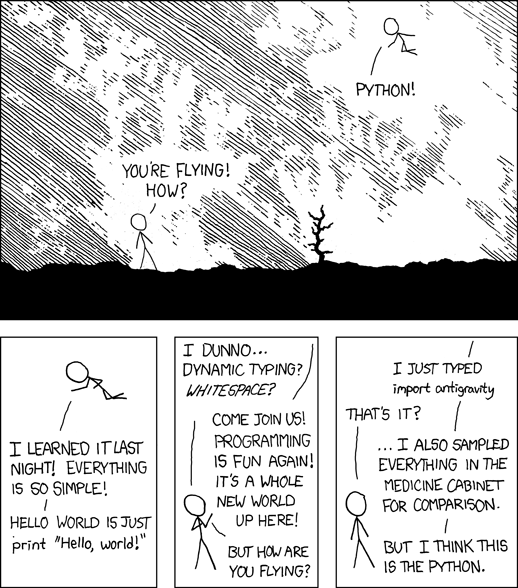# Data manipulation import pickle import pandas as pd import numpy as np # Machine Learning import sklearn import sklearn.ensemble import sklearn.svm from sklearn.cross_validation import train_test_split from sklearn.grid_search import GridSearchCV from sklearn.metrics import confusion_matrix from sklearn.metrics import classification_report # Plot import matplotlib.pyplot as plt import seaborn as sns from pylab import rcParams %matplotlib inline %config InlineBackend.figure_format='retina' rcParams['figure.figsize'] = 8, 5.5 # Utility functions from sklearn.metrics import roc_curve, auc from sklearn.preprocessing import label_binarize # Plot a confusion matrix def plotConfMap(confMat, classes=[], relative=False): width = len(confMat) height = len(confMat) oldParams = rcParams['figure.figsize'] rcParams['figure.figsize'] = width, height fig = plt.figure() plt.clf() plt.grid(False) ax = fig.add_subplot(111) ax.set_aspect(1) if not relative: res = ax.imshow(confMat, cmap='coolwarm', interpolation='nearest') else: res = ax.imshow(confMat, cmap='coolwarm', interpolation='nearest', vmin=0, vmax=100) for x in xrange(width): for y in xrange(height): ax.annotate(str(np.round(confMat[x][y], 1)), xy=(y, x), horizontalalignment='center', verticalalignment='center') fig.colorbar(res) if len(classes) > 0: plt.xticks(range(width), classes) plt.yticks(range(height), classes) rcParams['figure.figsize'] = oldParams return fig # Plot CV scores of a 2D grid search def plotGridResults2D(x, y, x_label, y_label, grid_scores): scores = [s for s in grid_scores] scores = np.array(scores).reshape(len(x), len(y)) plt.figure() plt.imshow(scores, interpolation='nearest', cmap=plt.cm.RdYlGn) plt.xlabel(y_label) plt.ylabel(x_label) plt.colorbar() plt.xticks(np.arange(len(y)), y, rotation=45) plt.yticks(np.arange(len(x)), x) plt.title('Validation accuracy') # Plot CV scores of a 1D "grid" search (a very narrow "grid") def plotGridResults1D(x, x_label, grid_scores): scores = np.array([s for s in grid_scores]) plt.figure() plt.plot(scores) plt.xlabel(x_label) plt.ylabel('Score') plt.xticks(np.arange(len(x)), x, rotation=45) plt.title('Validation accuracy')  ## Load and split dataset First, let’s load the dataset from our pickle file and split it into 3 sets: • learn for the learning phase, split into: • train for the training (will be used as validation with CV too) • test to evaluate the results • final for the final prediction, the one we will send to Kaggle # Load X_ids, X, y = pickle.load(open('Xy.pkl', 'rb')) y = y.reshape(y.shape) X_ids = np.array(X_ids) # Split learn and final with outcome indices i_final = (y == -1) i_learn = (y > -1) X_ids_final = X_ids[i_final] X_ids_learn = X_ids[i_learn] X_final = X[i_final, :] X_learn = X[i_learn, :] y_learn = y[i_learn] # Split train and test X_train, X_test, y_train, y_test = train_test_split(X_learn, y_learn, test_size=.25)  ## RBF SVM Having been taught Machine Learning in a large part by a researcher in SVM and kernel methods, my first Machine Learning try on a problem is often to use a SVM. ### Tuning the hyperparameters To optimize the hyperparameters of my SVM, I’m going to do a double step grid search, first looking at the optimal value on a coarse grid, and then on a more fine grid around a promising area. You can actually do this a lot better, and especially you can accelerate the coarse grid a lot (that’s supposed to be the idea of it being coarse) for example by using a subset of the dataset. Here we might have dropped a lot of non-bots bidders I think. But anyway, that’s how it is… One important thing is of course to think about finding the optimal value considering the AUC a scoring metrics, and not the classification rate. This obviously increase a lot your performance. I chose to use 10 CV because my “bot” class contains really not a lot of values and I don’t want to drop to much of them in the test fold. # Coarse grid C_range = np.r_[np.logspace(-2, 20, 13)] gamma_range = np.r_[np.logspace(-9, 5, 15)] grid = GridSearchCV(sklearn.svm.SVC(C=1.0, kernel='rbf', class_weight='auto', verbose=False, max_iter=60000), {'C' : C_range, 'gamma': gamma_range}, scoring='roc_auc', cv=10, n_jobs=8) grid.fit(X_learn, y_learn) plotGridResults2D(C_range, gamma_range, 'C', 'gamma', grid.grid_scores_) plt.show() # Display result C_best = np.round(np.log10(grid.best_params_['C'])) gamma_best = np.round(np.log10(grid.best_params_['gamma'])) print 'best C coarse:', C_best print 'best gamma coarse:', gamma_best # Fine grid C_range2 = np.r_[np.logspace(C_best - 1.5, C_best + 1.5, 15)] gamma_range2 = np.r_[np.logspace(gamma_best - 1.5, gamma_best + 1.5, 15)] gridFine = GridSearchCV(sklearn.svm.SVC(C=1.0, kernel='rbf', class_weight='auto', verbose=False, max_iter=60000), {'C' : C_range2, 'gamma': gamma_range2}, scoring='roc_auc', cv=10, n_jobs=8) gridFine.fit(X_learn, y_learn) plotGridResults2D(C_range2, gamma_range2, 'C', 'gamma', gridFine.grid_scores_) # Final result bestClf = gridFine.best_estimator_ bestClf.probability = True print bestClf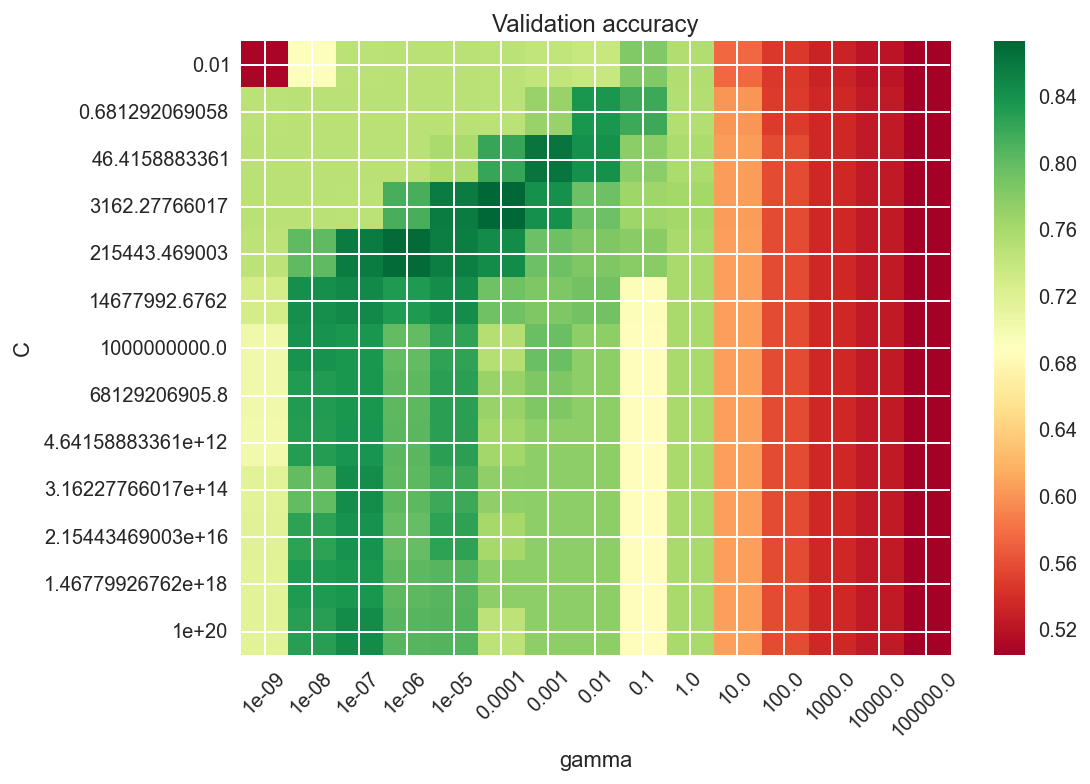best C coarse: 4.0 best gamma coarse: -4.0 SVC(C=517.94746792312128, cache_size=200, class_weight='auto', coef0=0.0, degree=3, gamma=0.00043939705607607906, kernel='rbf', max_iter=60000, probability=True, random_state=None, shrinking=True, tol=0.001, verbose=False)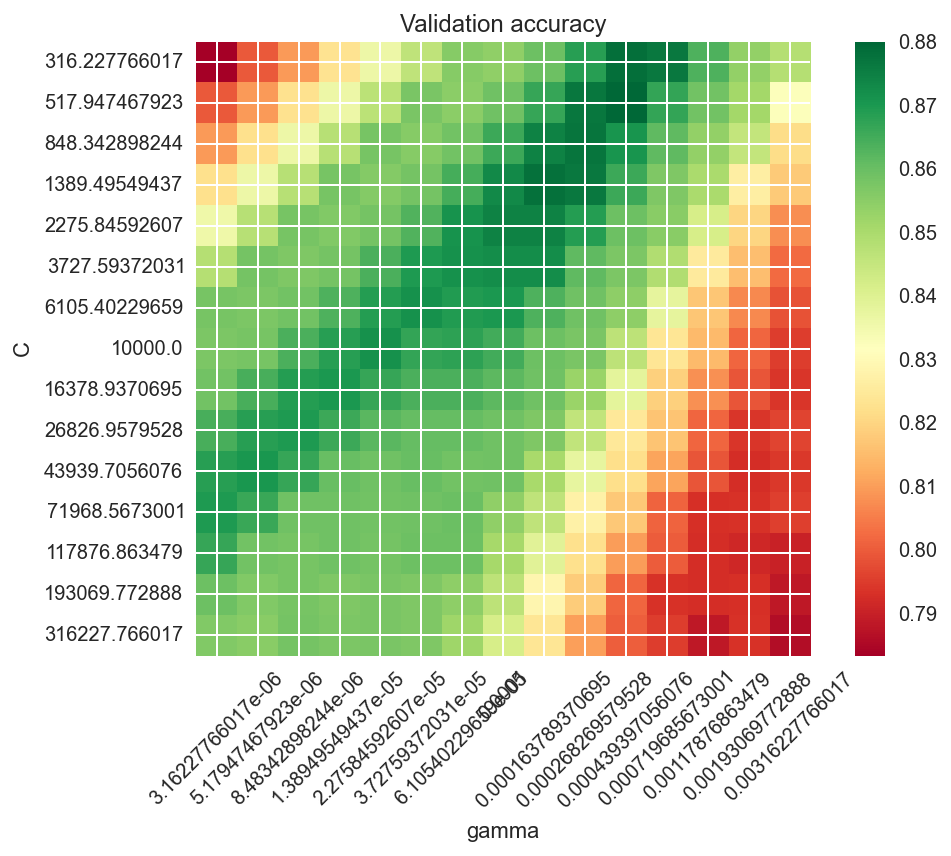### Testing the model Now that we have our CV-optimized hyperparameters, let’s learn this classifier on the full train set and evaluate it on the test set. # Fit it bestClf.fit(X_train, y_train) y_pred = bestClf.predict(X_test) # Classif report and conf mat print sklearn.metrics.classification_report(y_test, y_pred) plotConfMap(sklearn.metrics.confusion_matrix(y_test, y_pred)) plt.show() # Predict scores y_score = bestClf.decision_function(X_test) # Plot ROC fpr, tpr, _ = roc_curve(y_test, y_score) roc_auc = auc(fpr, tpr) plt.figure() plt.plot(fpr, tpr, label='ROC curve (area = %0.2f)' % roc_auc) plt.plot([0, 1], [0, 1], 'k--') plt.xlim([0.0, 1.0]) plt.ylim([0.0, 1.05]) plt.xlabel('False Positive Rate') plt.ylabel('True Positive Rate') plt.title('Receiver operating characteristic') plt.legend(loc="lower right") plt.show()   precision recall f1-score support 0.0 0.97 0.82 0.89 464 1.0 0.20 0.62 0.30 32 avg / total 0.92 0.81 0.85 496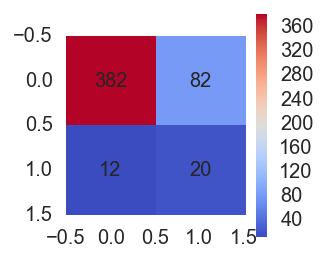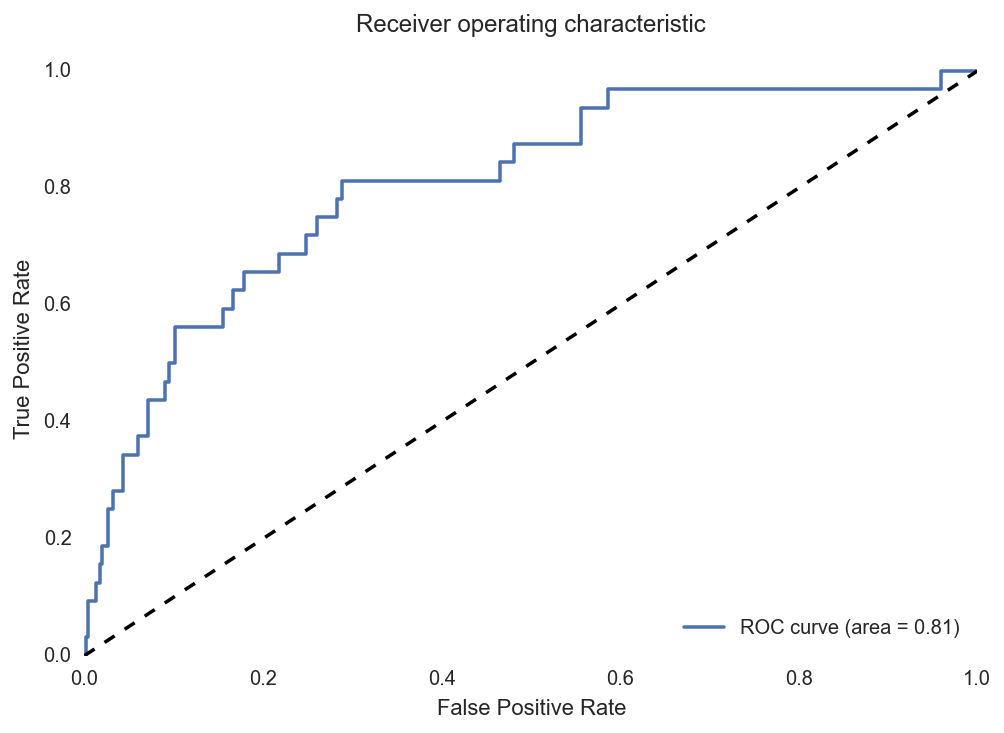### Final prediction Ok, now let’s make our final prediction, learing on the full learning set and prediction on the final set. # Fit on learn set and predict final set bestClf.fit(X_learn, y_learn) y_final = bestClf.predict_proba(X_final)[:,1] # Dirty join with test.csv to order them like it was before... # ... now that I look at this I am quite sure that Kaggle don't need them in the right # order so all this is actually pretty stupid. Sorry :) bidders_y_final = pd.DataFrame(np.c_[X_ids[i_final], y_final], columns=['bidder_id', 'prediction']) bidders_y_final[['prediction']] = bidders_y_final[['prediction']].astype(float) bidders_list = pd.read_csv('test.csv', header=0) bidders_list_final = pd.merge(bidders_list[['bidder_id']], bidders_y_final, how='left').fillna(0.0) # Write results to file f = open('predictions_RBF_SVM.csv', 'wb') f.write(bidders_list_final.to_csv(index=False)) f.close()  ## Linear L1 SVM Because of all the categories we kept from bids (highest frequent category use by a bidder, for a few variables), we have a lot of binary features, so it makes sense to use some L1 regularization. However, scikit-learn’s RBF SVM doesn’t have this feature, and I was too lazy to implement it myself so I tried the linear L1 SVM of scikit-learn. Also I wanted to try linear SVM as well. The code is basically the same as for RBF SVM. ### Tuning the hyperparameters # Coarse grid C_range = np.r_[np.logspace(-2, 10, 12)] grid = GridSearchCV(sklearn.svm.LinearSVC(C=1.0, penalty='l1', class_weight='auto', dual=False, verbose=False, max_iter=3000), {'C' : C_range}, cv=10, n_jobs=8, scoring='roc_auc') grid.fit(X_learn, y_learn) plotGridResults1D(C_range, 'C', grid.grid_scores_) plt.show() # Results C_best = np.round(np.log10(grid.best_params_['C'])) print 'best C coarse:', C_best # Fine grid C_range2 = np.r_[np.logspace(C_best - 1.5, C_best + 1.5, 15)] gridFine = GridSearchCV(sklearn.svm.LinearSVC(C=1.0, class_weight='auto', dual=False, verbose=False, max_iter=3000), {'C' : C_range2}, cv=10, n_jobs=8, scoring='roc_auc') gridFine.fit(X_learn, y_learn) plotGridResults1D(C_range2, 'C', gridFine.grid_scores_) # Final results bestClf = gridFine.best_estimator_ bestClf.probability = True print bestClf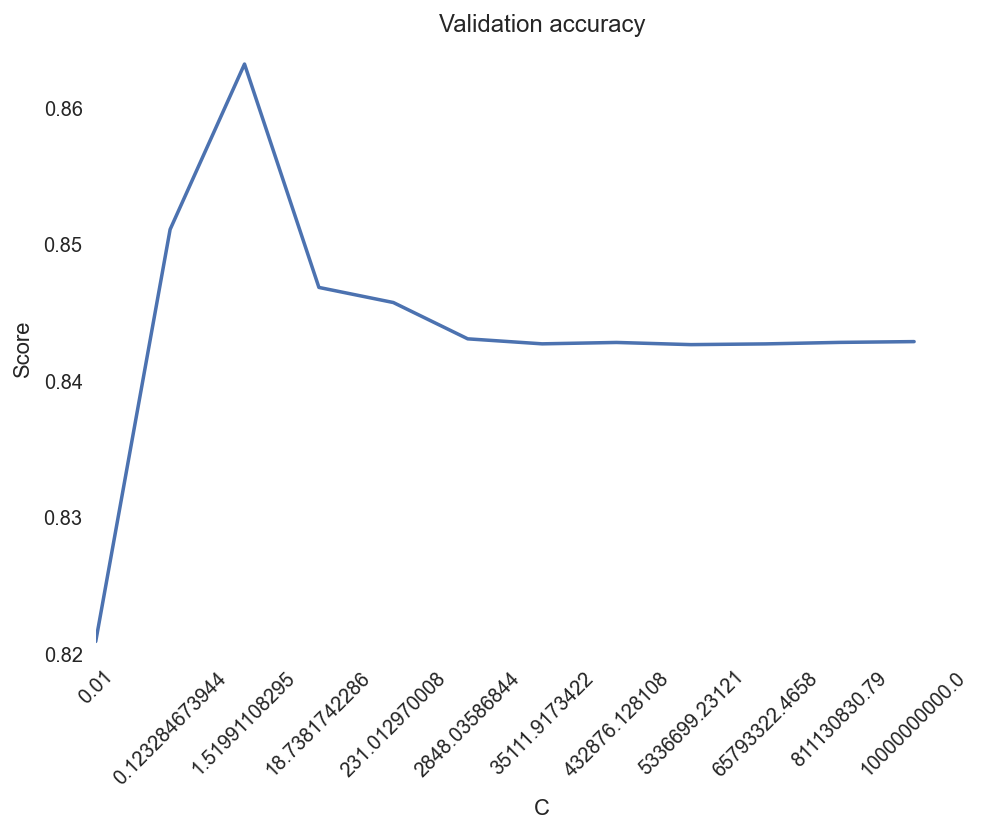best C coarse: 0.0 LinearSVC(C=0.13894954943731375, class_weight='auto', dual=False, fit_intercept=True, intercept_scaling=1, loss='squared_hinge', max_iter=3000, multi_class='ovr', penalty='l2', random_state=None, tol=0.0001, verbose=False)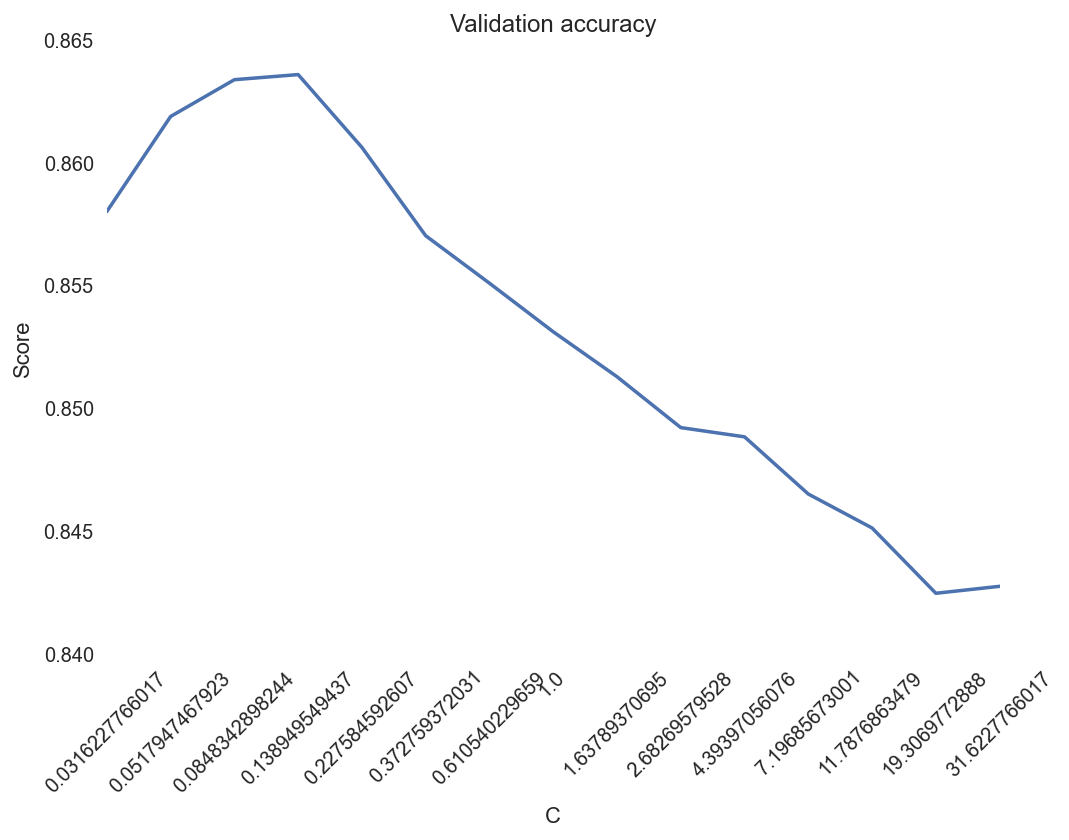### Testing the model # Fit on train bestClf.fit(X_train, y_train) y_pred = bestClf.predict(X_test) # Classification report print sklearn.metrics.classification_report(y_test, y_pred) plotConfMap(sklearn.metrics.confusion_matrix(y_test, y_pred)) plt.show() # Predict y_score = bestClf.decision_function(X_test) # Plot ROC fpr, tpr, _ = roc_curve(y_test, y_score) roc_auc = auc(fpr, tpr) plt.figure() plt.plot(fpr, tpr, label='ROC curve (area = %0.2f)' % roc_auc) plt.plot([0, 1], [0, 1], 'k--') plt.xlim([0.0, 1.0]) plt.ylim([0.0, 1.05]) plt.xlabel('False Positive Rate') plt.ylabel('True Positive Rate') plt.title('Receiver operating characteristic') plt.legend(loc="lower right") plt.show()   precision recall f1-score support 0.0 0.98 0.80 0.88 464 1.0 0.19 0.72 0.31 32 avg / total 0.93 0.79 0.84 496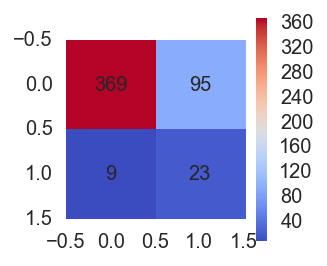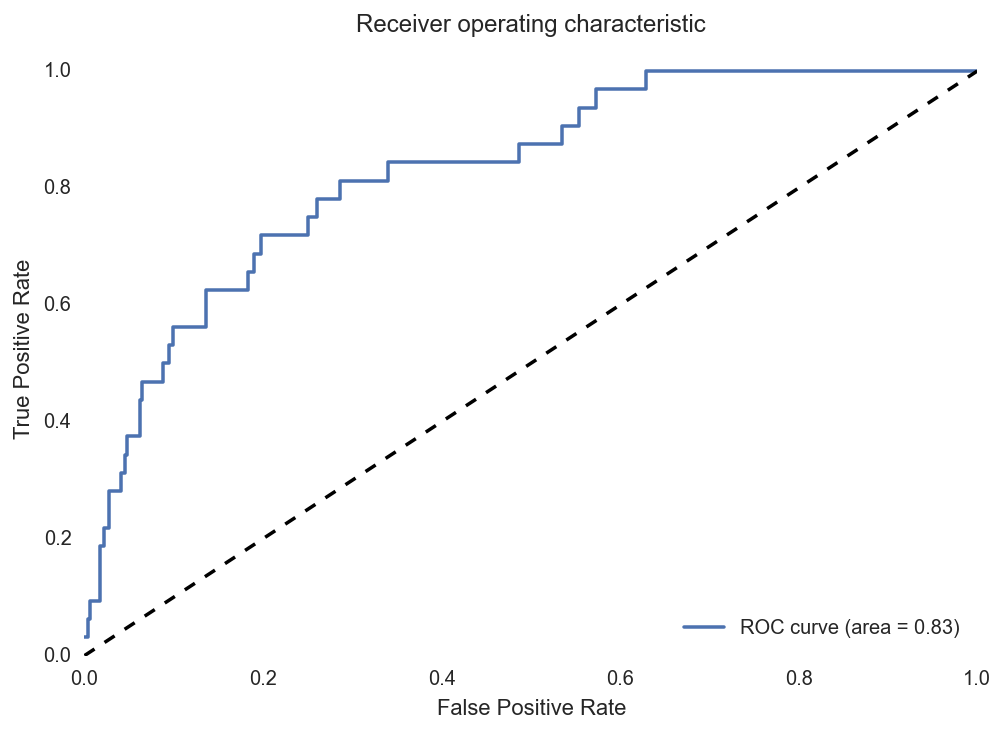### Final prediction # Fit on learn bestClf.fit(X_learn, y_learn) y_final = bestClf.predict(X_final) # Reorder prediction bidders_y_final = pd.DataFrame(np.c_[X_ids[i_final], y_final], columns=['bidder_id', 'prediction']) bidders_y_final[['prediction']] = bidders_y_final[['prediction']].astype(float) bidders_list = pd.read_csv('test.csv', header=0) bidders_list_final = pd.merge(bidders_list[['bidder_id']], bidders_y_final, how='left').fillna(0.0) # Save to file f = open('predictions_Lin_SVM_L1.csv', 'wb') f.write(bidders_list_final.to_csv(index=False)) f.close()  ## Random forest Also a classifier well known to be great is the random forest. The problem is that it can have a lot of hyperparameters to tune. Basically all the parameters in the trees plus the ensemble settings such as number of estimators, but also random feature subspace size if you want, etc. I chose to only CV grid search the number of estimators and the max depth of the tree. The idea of this code is again very close to the one of SVMs. But in this case I only used one grid search. If I wanted a perfect there would definitely be things to factorize between those different classifiers (and scikit-learn is actually very good for this with their consistant interfaces across classifiers). ### Tuning the hyperparameters # Coarse grid HP_range = np.array([1, 2, 3, 4, 5, 6, 7, 8, 9, 10, 12, 15, 18, 20, 25, 30, 50, 100]) HP2_range = np.array([11, 21, 35, 51, 75, 101, 151, 201, 251, 301, 351, 401, 451, 501]) grid = GridSearchCV(sklearn.ensemble.RandomForestClassifier(n_estimators=300, max_depth=None, max_features='auto', class_weight='auto'), {'max_depth' : HP_range, 'n_estimators' : HP2_range}, cv=sklearn.cross_validation.StratifiedKFold(y_learn, 5), n_jobs=8, scoring='roc_auc') grid.fit(X_learn, y_learn) plotGridResults2D(HP_range, HP2_range, 'max depth', 'n estimators', grid.grid_scores_) plt.show() # Final res bestClf = grid.best_estimator_ print bestClf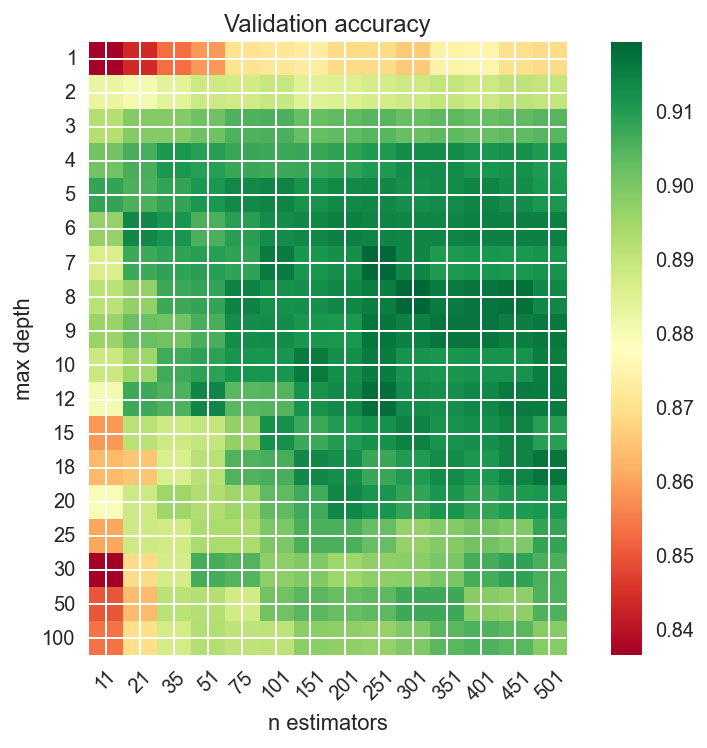RandomForestClassifier(bootstrap=True, class_weight='auto', criterion='gini', max_depth=8, max_features='auto', max_leaf_nodes=None, min_samples_leaf=1, min_samples_split=2, min_weight_fraction_leaf=0.0, n_estimators=301, n_jobs=1, oob_score=False, random_state=None, verbose=0, warm_start=False)  ### Testing the model # Ok, I actally want to choose these paramters myself! I'm god here, I do what I want! bestClf = sklearn.ensemble.RandomForestClassifier(max_depth=20, n_estimators=301, max_features='auto', class_weight='auto') # Learn on train for test bestClf.fit(X_train, y_train) y_pred = bestClf.predict(X_test) # Classification report print sklearn.metrics.classification_report(y_test, y_pred) plotConfMap(sklearn.metrics.confusion_matrix(y_test, y_pred)) plt.show() # Predict scores y_score = bestClf.predict_proba(X_test)[:,1] # ROC fpr, tpr, _ = roc_curve(y_test, y_score) roc_auc = auc(fpr, tpr) plt.figure() plt.plot(fpr, tpr, label='ROC curve (area = %0.2f)' % roc_auc) plt.plot([0, 1], [0, 1], 'k--') plt.xlim([0.0, 1.0]) plt.ylim([0.0, 1.05]) plt.xlabel('False Positive Rate') plt.ylabel('True Positive Rate') plt.title('Receiver operating characteristic') plt.legend(loc="lower right") plt.show()   precision recall f1-score support 0.0 0.94 1.00 0.97 464 1.0 0.83 0.16 0.26 32 avg / total 0.94 0.94 0.93 496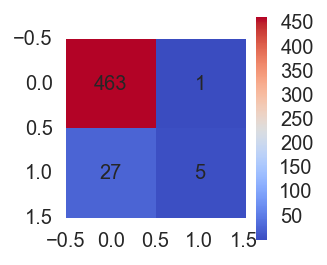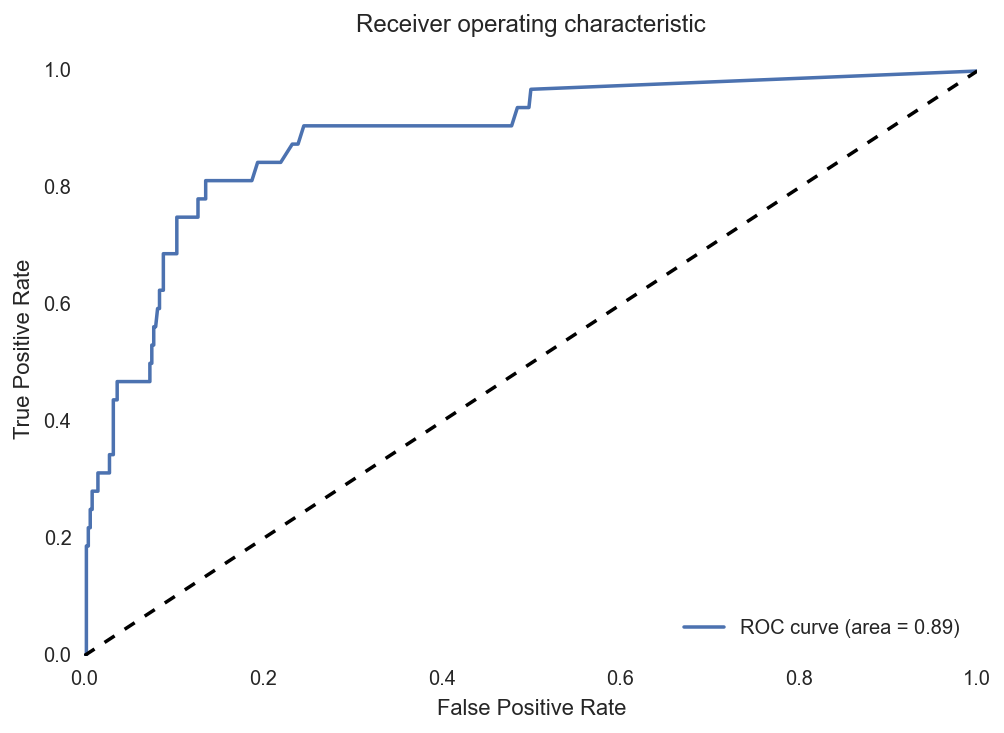bestClf.fit(X_learn, y_learn) y_final = bestClf.predict_proba(X_final)[:,1] bidders_y_final = pd.DataFrame(np.c_[X_ids[i_final], y_final], columns=['bidder_id', 'prediction']) bidders_y_final[['prediction']] = bidders_y_final[['prediction']].astype(float) bidders_list = pd.read_csv('test.csv', header=0) bidders_list_final = pd.merge(bidders_list[['bidder_id']], bidders_y_final, how='left').fillna(0.0) f = open('predictions_RF_2.0.csv', 'wb') f.write(bidders_list_final.to_csv(index=False)) f.close()  ## AdaBoost Ok I also tried AdaBoost which is also a nice ensemble technique but it didn’t gave particularly better results than random forest. I think you got the idea of my code so I spare you the code for AdaBoost… # Conclusion I finally submitted predictions of my random forest for evaluation on the private leaderboard. I think this competition was more about feature engeneering than the pure Machine Learning part. There are a lot of things I could have improved in my work. First, I could have used more complex features. Some good examples can be found for example on Kaggle’s forum of the competition “share your secret sauce” thread, or other similar threads. I think more complex time series analysis could have been done on bids times. I did not tried to clean the dataset, but I think that removing some wierd points from the training dataset might have been a good thing. Also I think there might be interesting ways to deal with the imbalance of the dataset (other than class weighting that I did of course), but I didn’t found time to dig into this. Finally regarding the pure Machine Learning part, using ensemble of different classifiers like some people did could also have improved the results. Anyway, I am quite surprised of the good results I got with so simple features and model compared to what some other people did. ## LogAnalysis: open-source web tool for Geographic Business Intelligence# Context I studied ASI (Architecture of Information Systems) at the INSA of Rouen, and during the second semester of the 4th year and the first semester of the 5th year, students work on a big R&D-like industrial project (PIC, for INSA Certified Project) for a company, carried out by teams of students. 5 projects are realized by 6 teams of 7 to 9 students during 1 scholar year, at a pace of 25 to 28 hours per week. One of those team work as a subcontractor for another team, and this team only exist during the first half of the project. # Subject The subject my team worked on was to make an Open Source web tool allowing each and every one to be able to do Geographical Business Intelligence (GeoBI) as easily as possible, without prior knowledge of the “theory” of GeoBI. This subject was proposed by our client, the Public Research Center Henri Tudor, based in Luxembourg, now called Luxembourg Institute for Science and Technology (LIST). The subcontractor team worked with mine on this subject. # Geographical Business Intelligence To summarize quickly, Business Intelligence (BI) allows you to analyze big quantities of data, usually structured around lots of dimensions. For example revenue as a function of time, geography, clients, business sector, products, etc. Business Intelligence allows you to analyze these masses of data, allowing you to make up your decisions based on those. We can split Business Intelligence into 3 big steps: 1. Collection of data to constitute a structured database 2. Selection and processing of data 3. Visualization of the results of the data processing ## Collection of data This preliminary step of Business Intelligence was not part of the project and is specific to each Information System. The goal is to collect data coming from multiple sources in order to constitute the most accurate and complete database possible. These data are stored in data warehouses and data marts. They will constitute OLAP databases. ## Selection and processing of data The first step of any BI analysis is to select which part of the data is interesting regarding the study you want to do (e.g.: select data for France in 2014 only). This is an OLAP slice. Then, the most common data processing is to aggregate the data to get the most relevant results (e.g.: aggregate by month and department). This is an OLAP dice. This is – in a very simplistic way of course – the principle of OLAP: limit the range of data studied and aggregate those at the most relevant level. ## Visualization of the results The second step of a BI analysis is to render the data for the user. The easiest display is of course the table. However, it is not the most practical way to interpret the data. We therefore use graphics, choropleth map, etc. # Goals of the project & demonstration The project is developed as an Open Source web interface that integrates into the already existing web app GeoNode, it is available on GitHub. The main goals of the project were to: • Load and process data using the OLAP database GeoMondrian • Display results on various charts: choropleth map, pie chart, bar chart, bubble chart, table, word cloud • Allow interaction on the charts to let the user query the OLAP database and do BI analyses: • Filtering (OLAP slice) by clicking • Dimensional zooming (OLAP drill-down and roll-up allowing to change both slice and dice) by mouse scroll. For example, a drill-down on France will display data for regions of France. Different variations of drill-down are available: simple (on 1 element), multiple (on more than 1 element), partial (drill-down while keeping elements of the upper level to compare data of various levels) Here is a quick demo of the final project that will help you visualize these goals more easily: # Architecture ## General overview On the following diagram, you can see the architecture of the solution developed:You can see the 4 main components: • The web interface, which role is to data visualization. It is composed of the packages: • analytics.js, a package developed that handles all the interface • QueryAPI, a proxy of the interfaces of Mandoline, the SOLAP API • crossfilter, a sort of small client side OLAP database • crossfilter server, a library we developed which allows us to do the same thing as we do with crossfilter, but delegate the OLAP computation to a SOLAP API for server side computation • d3.js, a library for SVG renderings of charts • dc.js, a modified version of the original dc.js for charts rendering of multidimensional crossfilter data • The web app in Python, analytics, delivering the data visualization interface, extending the existing web app GeoNode • The SOLAP API in Java called Mandoline, with its dependency olap4j, allowing us to query GeoMondrian easily in JSON • The OLAP database with a GeoMondrian SOLAP server getting data from a PostgreSQL database, doing the data processing ## analytics.js The analytics.js package represent the major part of the work that was done in this project. It is composed of various “namespaces”, each containing multiple “classes”. Of course, all this is JavaScript so “namespace” and “classes” are both objects. Here is the architecture of the package:You can see: • analytics.query simplifies the interrogation of the SOLAP API through QueryAPI by offering specialized functions • analytics.data offers data types representing OLAP elements and stores the data • analytics.state stores and gives functions to modify the OLAP state of the current analysis (to simplify, which elements of which level of which dimensions are analyzed) • analytics.display handles the interface (where is each chart and what is it displaying) and the interactions with the interface • analytics.charts offers charts classes that can be instantiated when you want to create a chart ## Cross-filtering and crossfilter-server.js One of the major “issue” we had to tackle was to provide an efficient cross-filtering on the interface. You can see this in the demonstration video above. Indeed, the filtering function is very useful for the user to be able to see how data are distributed across the elements of each dimension and explore the OLAP cube. This filtering function will therefore be highly solicited and should be very efficient while still being able to handle big volumes of data. There are actually two solutions to achieve this goal that can be represented on the following diagram:On this diagram, you can see the OLAP cube stored on the server on the left, and the charts we need to display on the right. ### First solution: Crossfilter The first solution is to ask our OLAP server, GeoMondrian, to compute a smaller cube of data containing what we are currently studying. Here on the diagram, the cube in the middle contains data for French regions, years and mode of transportation. This temporary cube is downloaded in the browser of the user and is then given to the library crossfilter. Crossfilter will then be capable of computing projections of the cube on each dimension, and these projections will be used by dc.js to display the charts. In this solution, the interface will be very responsive when filtering, because crossfilter already has got all the data he need to compute the projections while taking filters into account. It will take less than a second to compute new projections taking new filters into account. However, the defect of this solution is that the number of values in the temporary cube is the product of the number of elements studied in each dimension (e.g. for 6 regions, 2 years, 3 mode of transport we have 6 × 2 × 3 = 36 values), and this size grows exponentially. We will only support reasonable volumes because data will eventually become too big to be loaded in a reasonable time. ### Second solution: Crossfilter Server The alternative solution would be to ask GeoMondrian to directly compute the projection of the OLAP cube, after filtering, on each dimension studied, without computing the temporary cube that is too big. In that case, the size of the data we load is the sum of the number of elements in the dimensions we study (e.g. for 6 regions, 2 years, 3 mode of transport we have 6 + 2 + 3 = 11 values), and this size grows linearly. We will be able to support big volumes because we load much fewer data (as long as GeoMondrian can process those volumes of course). However, the drawback of this solution is that when the user changes the filters, we need to ask GeoMondrian to compute the projections on each dimension again, taking into account the new filters. This will significantly slow down the interface at each filtering because we need to query the server again while crossfilter only needed to do a few computations locally. Another issue with this solution is that our chart library, dc.js, is depending on Crossfilter as a data model. We can’t just give it some data. To solve this problem and make this alternative solution possible, we developed a small library that we called Crossfilter Server. This library has almost the same I/O interfaces as Crossfilter (only the initialization should differ). And Crossfilter Server will be able to reproduce Crossfilter’s behavior without having the full temporary cube, and by delegating all the computation to an API that will, in our case, call GeoMondrian. Therefore, we can either use dc.js with Crossfilter or Crossfilter Server. ### Recap & final solution To recap, we have 2 possibilities:Crossfilter •\mathcal{O}(n^p)\left(\prod_{i \in \mathcal{D}} n_i\right)\Rightarrow$Resonable volumes • Quick client side filtering & aggregates (< 10 ms)$\Rightarrow$Great responsivity Crossfilter Server •$\mathcal{O}(n\times p)\left(\sum_{i \in \mathcal{D}} n_i\right)\Rightarrow$Important volumes • Server side filtering & aggregates, reload data ($\approx$1s)$\Rightarrow$limited responsivity Our final solution was to use both of these solutions, using one or the other depending on the volume of data you are studying, and switching dynamically from one to the other when needed. # Conclusion This article is of course a quick summary of a very long project. If you want to know more about this project, you can read the documentation available in the wiki of the GitHub repository and take a look at the code of the components listed above. If you want, you can even contribute to the project by making pull-requests to it. ## Seventeen Equations That Changed The WorldI just finished the excellent book Seventeen Equations That Changed The World by Ian Stewart! From the title, you might think that this book will describe a few equations. It’s actually a lot more. Each equation is actually just a pretext to present a period of time and / or a field of mathematics related the equation. Don’t expect any important calculus in this book, it’s all about historical scientific context and usage. And it was actually a good thing for me. I knew and had used almost all the equations equations presented in this book, but there is actually not a lot of things that I knew from this book. The equations presented in the book are: Title Formula Author & Date Pythagoras Theorem$a^2+b^2=c^2$Pythagoras (530 BC) Logarithms$\log xy=\log x+\log y$John Napier (1610) Calculus$\frac{df}{dt}=\lim\limits_{h \to 0} \frac{f(t+h)-f(t)}{h}$Newton (1668) Law of Gravity$F=G\frac{m_1 m_2}{d^2}$Newton (1687) Imaginary Number$i^2=-1$Euler (1750) Euler’s Formula for Polyhedra$V-E+F=2$Euler (1751) Normal Distribution$\Phi(x) = \frac{1}{{\sigma \sqrt {2\pi } }}e^\frac {( x – \mu)^2 } {2\sigma ^2 }$C.F. Gauss (1810) Wave Equation$\frac{\partial ^2 u}{\partial t^2}=c^2\frac{\partial ^2 u}{\partial x^2}$J. d’Alembert (1746) Fourier Transform$f(\omega )=\int_{-\infty }^{+\infty }f(x) e^{-2\pi ix\omega }dx$J. Fourier (1822) Navier-Stokes Equation$\rho \left(\frac{\partial v}{\partial t}+v\cdot\triangledown v \right) = -\triangledown p + \triangledown\cdot T+f$C. Navier, G. Stoker (1845) Maxwell’s Equations$\triangledown\cdot E=0\triangledown\cdot H=0\triangledown \times E=-\frac{1}{c} \frac{\partial H}{\partial t}\triangledown \times H=\frac{1}{c}\frac{\partial E}{\partial t}$James Clerk Maxwell (1865) Second Law of Thermodynamics$dS\geq 0$Ludwig Boltzmann (1874) Relativity$E=mc^2$Albert Einstein (1905) Schrödinger’s Equation$i\hslash \frac{\partial }{\partial t} \psi=H\psi$Erwin Schrödinger (1927) Information Theory$H=-\sum p(x)\log p(x)$Claude Shannon (1949) Chaos Theory$x_{t+1}=kx_t(1-x_t)$Robert May (1975) Black-Scholes Equation$\frac{\sigma ^2}{2}S^2\frac{\partial ^2V}{\partial S^2}+rS\frac{\partial V}{\partial S}+\frac{\partial V}{\partial t}-rV=0$F. Black, M. Scholes (1990) As I said, it’s actually a lot more than about the equations. For example, the first chapter about Pythagoras theorem will of course present the history of this well known formula and some ideas about the proof of it. But a big part of the chapter is about the history of mathematical symbolism and how we went from the original Pythagoras theorem, that was a sentence, to the current notation$a^2 + b^2 = c^2\$, the difference between math and science knowledge at that time and math nowadays (for example about the shape of Earth). The chapter will also evoke all the mathematical field of geometry, mentioning Elements by Euclid, the definition of trigonometry and its usage for map making and astronomy for example, the existence of non-Euclidean geometry, and even more!

I would highly recommend you this book if you like science and/or engineering, and even if you’re not a particularly a big fan of math.

If you want a preview of the book, you can read an extract at Google Books or Amazon for example.